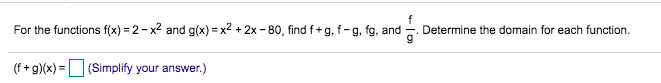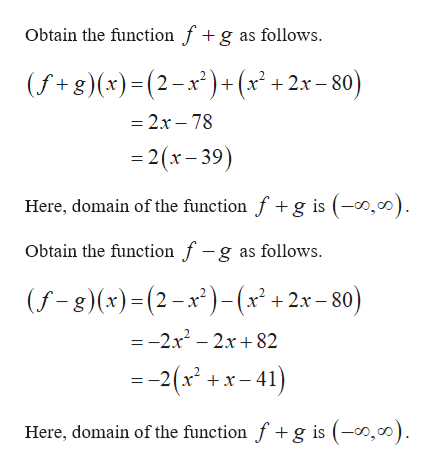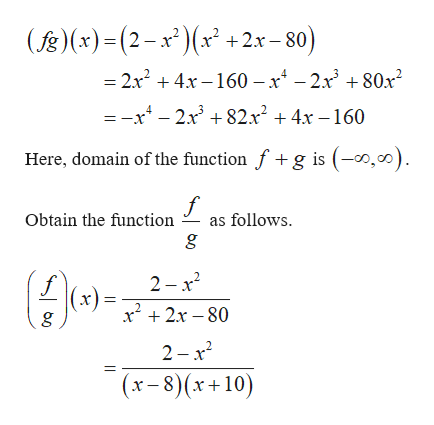# For the functions f(x) 2-x2 and g(x) x2 +2x -80, find f+ g, f- g, fg, andDetermine the domain for eaach function(f+g)(x)(Simplify your answer.)

Question
1 viewshelp_outlineImage TranscriptioncloseFor the functions f(x) 2-x2 and g(x) x2 +2x -80, find f+ g, f- g, fg, and Determine the domain for eaach function (f+g)(x)(Simplify your answer.) fullscreen
check_circle

Step 1

The given functions are f(x) = 2 - x2 and g(x)  = x2 + 2x - 80.help_outlineImage TranscriptioncloseObtain the function fg as follows (f+g)(x)(2-x)+(x +2x-80) =2x- 78 - 2(x-39) Here, domain of the function f+ g is (-0,00) Obtain the function f -g as follows. (f-8) (x)-(2-x*)-(x + 2x-80) =-2x2- 2x+82 2(x +x-41) Here, domain of the function f+g is -o,o) fullscreen
Step 2

Obtain the function  f...help_outlineImage Transcriptionclose((x)(2-x(x +2x-80) - 2x2 4x-160 - x4 - 2.x3 + 80x2 = -x4 - 2x3 +82x2 4x -160 Here, domain of the function f+ g is (-0,00) f as follows Obtain the function 2 x2 x2x 80 2 -x2 (x-8)(x+10) fullscreen

### Want to see the full answer?

See Solution

#### Want to see this answer and more?

Solutions are written by subject experts who are available 24/7. Questions are typically answered within 1 hour.*

See Solution
*Response times may vary by subject and question.
Tagged in

### Other